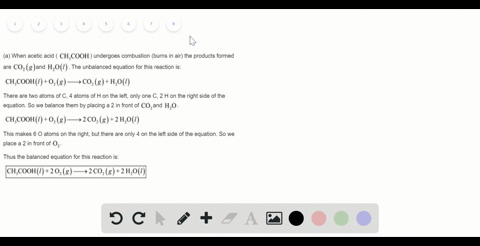Sign up for our free STEM online summer camps starting June 1st!View Summer Courses### Write the balanced chemical equations for (a) the…

01:43Auburn University Main Campus

Need more help? Fill out this quick form to get professional live tutoring.

Get live tutoring
Problem 86

When hydrogen sulfide gas is bubbled into a solution of sodium hydroxide, the reaction forms sodium sulfide and water. How many grams of sodium sulfide are formed if 1.25 $\mathrm{g}$ of hydrogen sulfide is bubbled into a solution containing 2.00 g of sodium hydroxide, assuming that the sodium sulfide is made in 92.0$\%$ yield?

See explanation

## Discussion

You must be signed in to discuss.

## Video Transcript

So here we have the balanced equation for the creation ofthe ideas, so fight with sodium hydroxide. So in the reaction condition we have given that one one two five grand off hydrogen sulphide is reacting with two gram of sodium hydroxide. We have to find out how many grams off sodium sulphide are present. Assuming that the person sealed off the relation is ninety two percent. So what we have to do first is we have to find out the number of mall self one point two five gram office to S and program off sodium hydroxide. And from there we'LL have to find out how many moles off Sorry himself fight will it will be produced. Ah, in theory or what is the theoretical ailed off sodium sulfide? And from there we'LL have to find out the actual field of sodium sulfide. So one point two five gram hydrogen sulphide means identical to a number of malls. It will be one point two five graham over the molar mass off H two s, which is parity for point one gram per mall and will give us a fellow off zero point zero formal and to Graham off sodium hydroxide in number of malls will be two gram over the molar mass of sodium hydroxide, which is for diagram Parman, and it will give us a value off the sirah point is zero five more. Now I have to find out which one is our limiting reacting because the product it will be based on the amount of limiting reactant. So when we're home from here, you can see that one more love is to us will react with to matloff sodium hydroxide that men's point zero formal office twist will reactivate point zero eight matloff sodium hydroxide. But we have point zero five full of sodium hydroxide. That means this is the reactant, which will be completely consumed because it is lower in amount than the required amount. That means this amount will be completely consumed. And there's a story. A Madras cereal is our limiting reactant, and we have tto calculate the critical ill of sodium sulphide, best on the amount of sodium hydroxide. So from here, you can see that tomb all of sodium hydroxide will produced will produce one more love story in sulfide that men's khun right tumult sodium hydroxide will produce one more sodium sulphide. That means point zero five mall sodium hydroxide will produce one over pull times zero point zero five more sodium sulphide R, which is equal to point zero two five more officer himself fight So this is the theoretical, sealed off sodium sulphide. But we're saying that actually, Phyllis ninety two percent that men's the actual product off in it, Wes will be zero point zero two five times ninety two percent R ninety two hundred and which will give us zero porn. Zero toe, three mall. So this is the actual end ofthe sodium sulphide produced. Now we will have to find out the mass for this much matloff sodium sulfide So zero point zero two three mall of sodium sulphide means zero point zero two three mall times The Moler mass of sodium sulphide, which is seventy. Eat grandpa, our mall, and it will give us hello off one burned seven nine graham. So one point sir, nine grams sodium sulphide will be will be found or farmed in this reaction condition